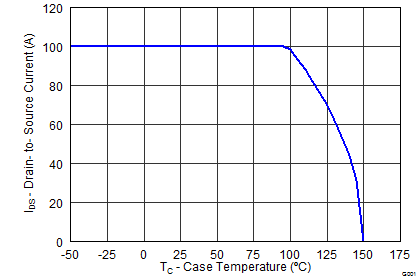ZHCSBW5B December   2013  – May 2017

PRODUCTION DATA.

1. 1特性
2. 2应用范围
3. 3说明
4. 4修订历史记录
5. 5Specifications
6. 6器件和文档支持
7. 7机械、封装和可订购信息

• DNK|8

## 5 Specifications

### 5.1 Electrical Characteristics

(TA = 25°C unless otherwise stated)
PARAMETER TEST CONDITIONS MIN TYP MAX UNIT
STATIC CHARACTERISTICS
BVDSS Drain-to-Source Voltage VGS = 0 V, ID = 250 μA 80 V
IDSS Drain-to-Source Leakage Current VGS = 0 V, VDS = 64 V 1 μA
IGSS Gate-to-Source Leakage Current VDS = 0 V, VGS = 20 V 100 nA
VGS(th) Gate-to-Source Threshold Voltage VDS = VGS, ID = 250 μA 2.2 2.7 3.3 V
RDS(on) Drain-to-Source On Resistance VGS = 6 V, ID = 19 A 3.8 4.8
VGS = 10 V, ID = 19 A 3.4 4.1
gfs Transconductance VDS = 8 V, ID = 19 A 88 S
DYNAMIC CHARACTERISTICS
Ciss Input Capacitance VGS = 0 V, VDS = 40 V, ƒ = 1 MHz 3750 4870 pF
Coss Output Capacitance 925 1202 pF
Crss Reverse Transfer Capacitance 17 22 pF
RG Series Gate Resistance 1.2 2.4 Ω
Qg Gate Charge Total (10 V) VDS = 40 V, ID = 19 A 48 62 nC
Qgd Gate Charge Gate to Drain 8.6 nC
Qgs Gate Charge Gate to Source 14 nC
Qg(th) Gate Charge at Vth 10 nC
Qoss Output Charge VDS = 40 V, VGS = 0 V 130 nC
td(on) Turn On Delay Time VDS = 40 V, VGS = 10 V,
IDS = 19 A, RG = 0 Ω
8 ns
tr Rise Time 6 ns
td(off) Turn Off Delay Time 22 ns
tf Fall Time 7 ns
DIODE CHARACTERISTICS
VSD Diode Forward Voltage ISD = 19 A, VGS = 0 V 0.8 1 V
Qrr Reverse Recovery Charge VDS= 40 V, IF = 19 A,
di/dt = 300 A/μs
275 nC
trr Reverse Recovery Time 72 ns

### 5.2 Thermal Information

(TA = 25°C unless otherwise stated)
THERMAL METRIC MIN TYP MAX UNIT
RθJC Junction-to-Case Thermal Resistance(1) 0.8 °C/W
RθJA Junction-to-Ambient Thermal Resistance (1)(2) 50
RθJC is determined with the device mounted on a 1-inch2 (6.45-cm2), 2-oz. (0.071-mm thick) Cu pad on a 1.5-inches × 1.5-inches (3.81-cm × 3.81-cm), 0.06-inch (1.52-mm) thick FR4 PCB. RθJC is specified by design, whereas RθJA is determined by the user’s board design.
Device mounted on FR4 material with 1-inch2 (6.45-cm2), 2-oz. (0.071-mm thick) Cu.Max RθJA = 50°C/W when mounted on 1 inch2 (6.45 cm2) of 2-oz. (0.071-mm thick) Cu.Max RθJA = 125°C/W when mounted on a minimum pad area of 2-oz. (0.071-mm thick) Cu.

### 5.3 Typical MOSFET Characteristics

(TA = 25°C unless otherwise stated)Figure 1. Transient Thermal ImpedanceFigure 2. Saturation CharacteristicsFigure 4. Gate ChargeFigure 6. Threshold Voltage vs TemperatureFigure 8. Normalized On-State Resistance vs TemperatureFigure 10. Maximum Safe Operating AreaFigure 12. Maximum Drain Current vs TemperatureFigure 3. Transfer CharacteristicsFigure 5. CapacitanceFigure 7. On-State Resistance vs Gate-to-Source VoltageFigure 9. Typical Diode Forward VoltageFigure 11. Single Pulse Unclamped Inductive Switching